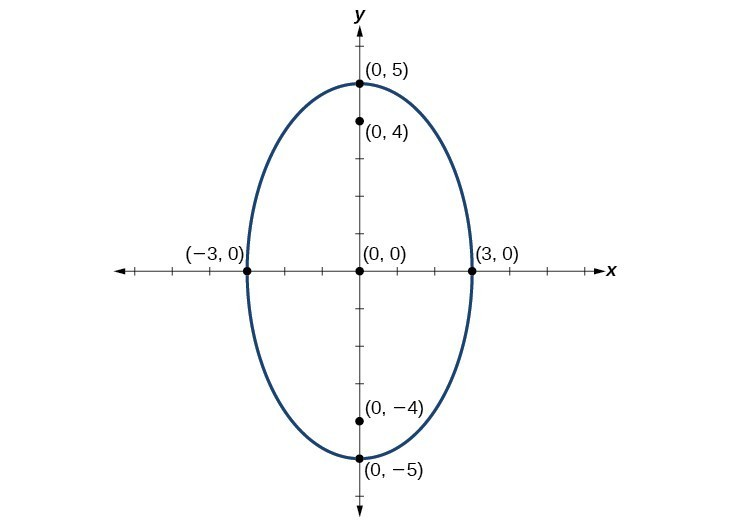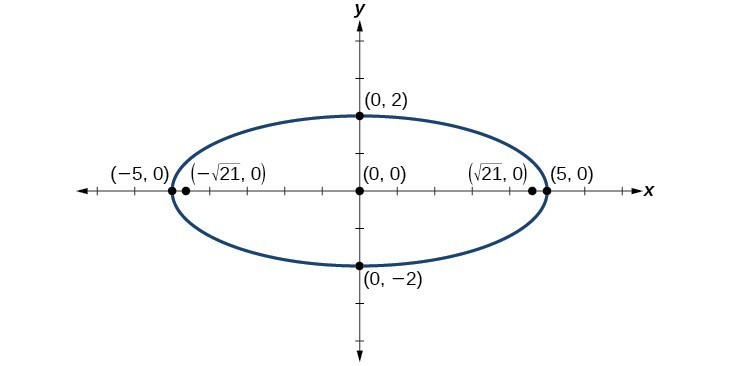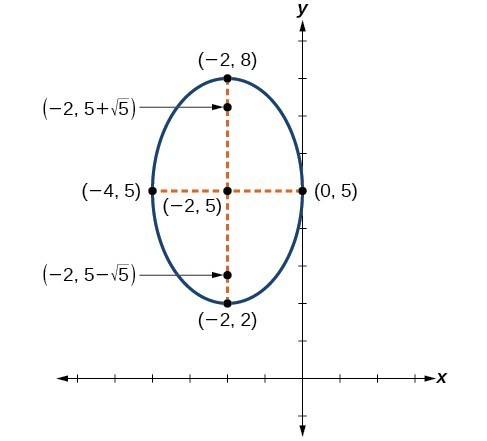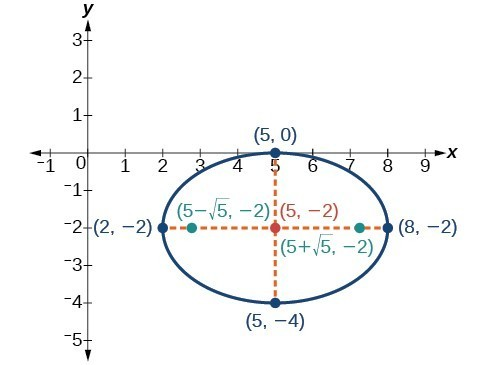## Graphing Ellipses

Just as we can write the equation for an ellipse given its graph, we can graph an ellipse given its equation. To graph ellipses centered at the origin, we use the standard form $\frac{{x}^{2}}{{a}^{2}}+\frac{{y}^{2}}{{b}^{2}}=1,\text{ }a>b$ for horizontal ellipses and $\frac{{x}^{2}}{{b}^{2}}+\frac{{y}^{2}}{{a}^{2}}=1,\text{ }a>b$ for vertical ellipses.

### How To: Given the standard form of an equation for an ellipse centered at $\left(0,0\right)$, sketch the graph.

• Use the standard forms of the equations of an ellipse to determine the major axis, vertices, co-vertices, and foci.
• If the equation is in the form $\frac{{x}^{2}}{{a}^{2}}+\frac{{y}^{2}}{{b}^{2}}=1$, where $a>b$, then
• the major axis is the x-axis
• the coordinates of the vertices are $\left(\pm a,0\right)$
• the coordinates of the co-vertices are $\left(0,\pm b\right)$
• the coordinates of the foci are $\left(\pm c,0\right)$
• If the equation is in the form $\frac{{x}^{2}}{{b}^{2}}+\frac{{y}^{2}}{{a}^{2}}=1$, where $a>b$, then
• the major axis is the y-axis
• the coordinates of the vertices are $\left(0,\pm a\right)$
• the coordinates of the co-vertices are $\left(\pm b,0\right)$
• the coordinates of the foci are $\left(0,\pm c\right)$
• Solve for $c$ using the equation ${c}^{2}={a}^{2}-{b}^{2}$.
• Plot the center, vertices, co-vertices, and foci in the coordinate plane, and draw a smooth curve to form the ellipse.

### Example 3: Graphing an Ellipse Centered at the Origin

Graph the ellipse given by the equation, $\frac{{x}^{2}}{9}+\frac{{y}^{2}}{25}=1$. Identify and label the center, vertices, co-vertices, and foci.

### Solution

First, we determine the position of the major axis. Because $25>9$, the major axis is on the y-axis. Therefore, the equation is in the form $\frac{{x}^{2}}{{b}^{2}}+\frac{{y}^{2}}{{a}^{2}}=1$, where ${b}^{2}=9$ and ${a}^{2}=25$. It follows that:

• the center of the ellipse is $\left(0,0\right)$
• the coordinates of the vertices are $\left(0,\pm a\right)=\left(0,\pm \sqrt{25}\right)=\left(0,\pm 5\right)$
• the coordinates of the co-vertices are $\left(\pm b,0\right)=\left(\pm \sqrt{9},0\right)=\left(\pm 3,0\right)$
• the coordinates of the foci are $\left(0,\pm c\right)$, where ${c}^{2}={a}^{2}-{b}^{2}$ Solving for $c$, we have:
$\begin{array}{l}c=\pm \sqrt{{a}^{2}-{b}^{2}}\hfill \\ =\pm \sqrt{25 - 9}\hfill \\ =\pm \sqrt{16}\hfill \\ =\pm 4\hfill \end{array}$

Therefore, the coordinates of the foci are $\left(0,\pm 4\right)$.

Next, we plot and label the center, vertices, co-vertices, and foci, and draw a smooth curve to form the ellipse.Figure 8

### Try It 3

Graph the ellipse given by the equation $\frac{{x}^{2}}{36}+\frac{{y}^{2}}{4}=1$. Identify and label the center, vertices, co-vertices, and foci.

Solution

### Example 4: Graphing an Ellipse Centered at the Origin from an Equation Not in Standard Form

Graph the ellipse given by the equation $4{x}^{2}+25{y}^{2}=100$. Rewrite the equation in standard form. Then identify and label the center, vertices, co-vertices, and foci.

### Solution

First, use algebra to rewrite the equation in standard form.

$\begin{array}{l} 4{x}^{2}+25{y}^{2}=100\hfill \\ \text{ }\frac{4{x}^{2}}{100}+\frac{25{y}^{2}}{100}=\frac{100}{100}\hfill \\ \text{ }\frac{{x}^{2}}{25}+\frac{{y}^{2}}{4}=1\hfill \end{array}$

Next, we determine the position of the major axis. Because $25>4$, the major axis is on the x-axis. Therefore, the equation is in the form $\frac{{x}^{2}}{{a}^{2}}+\frac{{y}^{2}}{{b}^{2}}=1$, where ${a}^{2}=25$ and ${b}^{2}=4$. It follows that:

• the center of the ellipse is $\left(0,0\right)$
• the coordinates of the vertices are $\left(\pm a,0\right)=\left(\pm \sqrt{25},0\right)=\left(\pm 5,0\right)$
• the coordinates of the co-vertices are $\left(0,\pm b\right)=\left(0,\pm \sqrt{4}\right)=\left(0,\pm 2\right)$
• the coordinates of the foci are $\left(\pm c,0\right)$, where ${c}^{2}={a}^{2}-{b}^{2}$. Solving for $c$, we have:
$\begin{array}{l}c=\pm \sqrt{{a}^{2}-{b}^{2}}\hfill \\ =\pm \sqrt{25 - 4}\hfill \\ =\pm \sqrt{21}\hfill \end{array}$

Therefore the coordinates of the foci are $\left(\pm \sqrt{21},0\right)$.

Next, we plot and label the center, vertices, co-vertices, and foci, and draw a smooth curve to form the ellipse.Figure 9

### Try It 4

Graph the ellipse given by the equation $49{x}^{2}+16{y}^{2}=784$. Rewrite the equation in standard form. Then identify and label the center, vertices, co-vertices, and foci.

Solution

## Graphing Ellipses Not Centered at the Origin

When an ellipse is not centered at the origin, we can still use the standard forms to find the key features of the graph. When the ellipse is centered at some point, $\left(h,k\right)$, we use the standard forms $\frac{{\left(x-h\right)}^{2}}{{a}^{2}}+\frac{{\left(y-k\right)}^{2}}{{b}^{2}}=1,\text{ }a>b$ for horizontal ellipses and $\frac{{\left(x-h\right)}^{2}}{{b}^{2}}+\frac{{\left(y-k\right)}^{2}}{{a}^{2}}=1,\text{ }a>b$ for vertical ellipses. From these standard equations, we can easily determine the center, vertices, co-vertices, foci, and positions of the major and minor axes.

### How To: Given the standard form of an equation for an ellipse centered at $\left(h,k\right)$, sketch the graph.

• Use the standard forms of the equations of an ellipse to determine the center, position of the major axis, vertices, co-vertices, and foci.
• If the equation is in the form $\frac{{\left(x-h\right)}^{2}}{{a}^{2}}+\frac{{\left(y-k\right)}^{2}}{{b}^{2}}=1$, where $a>b$, then
• the center is $\left(h,k\right)$
• the major axis is parallel to the x-axis
• the coordinates of the vertices are $\left(h\pm a,k\right)$
• the coordinates of the co-vertices are $\left(h,k\pm b\right)$
• the coordinates of the foci are $\left(h\pm c,k\right)$
• If the equation is in the form $\frac{{\left(x-h\right)}^{2}}{{b}^{2}}+\frac{{\left(y-k\right)}^{2}}{{a}^{2}}=1$, where $a>b$, then
• the center is $\left(h,k\right)$
• the major axis is parallel to the y-axis
• the coordinates of the vertices are $\left(h,k\pm a\right)$
• the coordinates of the co-vertices are $\left(h\pm b,k\right)$
• the coordinates of the foci are $\left(h,k\pm c\right)$
• Solve for $c$ using the equation ${c}^{2}={a}^{2}-{b}^{2}$.
• Plot the center, vertices, co-vertices, and foci in the coordinate plane, and draw a smooth curve to form the ellipse.

### Example 5: Graphing an Ellipse Centered at (h, k)

Graph the ellipse given by the equation, $\frac{{\left(x+2\right)}^{2}}{4}+\frac{{\left(y - 5\right)}^{2}}{9}=1$. Identify and label the center, vertices, co-vertices, and foci.

### Solution

First, we determine the position of the major axis. Because $9>4$, the major axis is parallel to the y-axis. Therefore, the equation is in the form $\frac{{\left(x-h\right)}^{2}}{{b}^{2}}+\frac{{\left(y-k\right)}^{2}}{{a}^{2}}=1$, where ${b}^{2}=4$ and ${a}^{2}=9$. It follows that:

• the center of the ellipse is $\left(h,k\right)=\left(-2,\text{5}\right)$
• the coordinates of the vertices are $\left(h,k\pm a\right)=\left(-2,5\pm \sqrt{9}\right)=\left(-2,5\pm 3\right)$, or $\left(-2,\text{2}\right)$ and $\left(-2,\text{8}\right)$
• the coordinates of the co-vertices are $\left(h\pm b,k\right)=\left(-2\pm \sqrt{4},5\right)=\left(-2\pm 2,5\right)$, or $\left(-4,5\right)$ and $\left(0,\text{5}\right)$
• the coordinates of the foci are $\left(h,k\pm c\right)$, where ${c}^{2}={a}^{2}-{b}^{2}$. Solving for $c$, we have:
$\begin{array}{l}\begin{array}{l}\\ c=\pm \sqrt{{a}^{2}-{b}^{2}}\end{array}\hfill \\ =\pm \sqrt{9 - 4}\hfill \\ =\pm \sqrt{5}\hfill \end{array}$

Therefore, the coordinates of the foci are $\left(-2,\text{5}-\sqrt{5}\right)$ and $\left(-2,\text{5+}\sqrt{5}\right)$.

Next, we plot and label the center, vertices, co-vertices, and foci, and draw a smooth curve to form the ellipse.Figure 10

### Try It 5

Graph the ellipse given by the equation $\frac{{\left(x - 4\right)}^{2}}{36}+\frac{{\left(y - 2\right)}^{2}}{20}=1$. Identify and label the center, vertices, co-vertices, and foci.

Solution

### How To: Given the general form of an equation for an ellipse centered at (h, k), express the equation in standard form.

• Recognize that an ellipse described by an equation in the form $a{x}^{2}+b{y}^{2}+cx+dy+e=0$ is in general form.
• Rearrange the equation by grouping terms that contain the same variable. Move the constant term to the opposite side of the equation.
• Factor out the coefficients of the ${x}^{2}$ and ${y}^{2}$ terms in preparation for completing the square.
• Complete the square for each variable to rewrite the equation in the form of the sum of multiples of two binomials squared set equal to a constant, ${m}_{1}{\left(x-h\right)}^{2}+{m}_{2}{\left(y-k\right)}^{2}={m}_{3}$, where ${m}_{1},{m}_{2}$, and ${m}_{3}$ are constants.
• Divide both sides of the equation by the constant term to express the equation in standard form.

### Example 6: Graphing an Ellipse Centered at (h, k) by First Writing It in Standard Form

Graph the ellipse given by the equation $4{x}^{2}+9{y}^{2}-40x+36y+100=0$. Identify and label the center, vertices, co-vertices, and foci.

### Solution

We must begin by rewriting the equation in standard form.

$4{x}^{2}+9{y}^{2}-40x+36y+100=0$

Group terms that contain the same variable, and move the constant to the opposite side of the equation.

$\left(4{x}^{2}-40x\right)+\left(9{y}^{2}+36y\right)=-100$

Factor out the coefficients of the squared terms.

$4\left({x}^{2}-10x\right)+9\left({y}^{2}+4y\right)=-100$

Complete the square twice. Remember to balance the equation by adding the same constants to each side.

$4\left({x}^{2}-10x+25\right)+9\left({y}^{2}+4y+4\right)=-100+100+36$

Rewrite as perfect squares.

$4{\left(x - 5\right)}^{2}+9{\left(y+2\right)}^{2}=36$

Divide both sides by the constant term to place the equation in standard form.

$\frac{{\left(x - 5\right)}^{2}}{9}+\frac{{\left(y+2\right)}^{2}}{4}=1$

Now that the equation is in standard form, we can determine the position of the major axis. Because $9>4$, the major axis is parallel to the x-axis. Therefore, the equation is in the form $\frac{{\left(x-h\right)}^{2}}{{a}^{2}}+\frac{{\left(y-k\right)}^{2}}{{b}^{2}}=1$, where ${a}^{2}=9$ and ${b}^{2}=4$. It follows that:

• the center of the ellipse is $\left(h,k\right)=\left(5,-2\right)$
• the coordinates of the vertices are $\left(h\pm a,k\right)=\left(5\pm \sqrt{9},-2\right)=\left(5\pm 3,-2\right)$, or $\left(2,-2\right)$ and $\left(8,-2\right)$
• the coordinates of the co-vertices are $\left(h,k\pm b\right)=\left(\text{5},-2\pm \sqrt{4}\right)=\left(\text{5},-2\pm 2\right)$, or $\left(5,-4\right)$ and $\left(5,\text{0}\right)$
• the coordinates of the foci are $\left(h\pm c,k\right)$, where ${c}^{2}={a}^{2}-{b}^{2}$. Solving for $c$, we have:
$\begin{array}{l}c=\pm \sqrt{{a}^{2}-{b}^{2}}\hfill \\ =\pm \sqrt{9 - 4}\hfill \\ =\pm \sqrt{5}\hfill \end{array}$

Therefore, the coordinates of the foci are $\left(\text{5}-\sqrt{5},-2\right)$ and $\left(\text{5+}\sqrt{5},-2\right)$.

Next we plot and label the center, vertices, co-vertices, and foci, and draw a smooth curve to form the ellipse.Figure 11

### Try It 6

Express the equation of the ellipse given in standard form. Identify the center, vertices, co-vertices, and foci of the ellipse.

$4{x}^{2}+{y}^{2}-24x+2y+21=0$